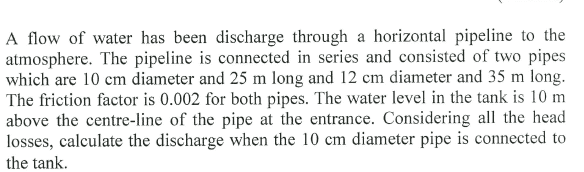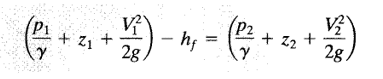# Pressure in pipe

## Homework Statement[/B]
in this question , the Pressure at entrance is not given , how to do this?

## The Attempt at a Solution

does the author mean the pressure at entrance = pressure at exit

billy_joule

## Homework Statement

in this question , the Pressure at entrance is not given ,
You are expected to find it from the depth of the tank.

You are expected to find it from the depth of the tank.
i am going to use this equation to solve this question , where z = 10 m what is the pressure at ENTRANCE ? it's not givenp/s : hf = 0

billy_joule